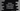# Python Tutorial : Part 7 : python tuplePython tuple:

Python tuple is same as python list but it is immutable. We cannot change a tuple like lists.

Where to use tuples:

• Iterating through touple is slightly faster than lists. If you have all constant set of values, use a tuple instead of a list.

• Since all data in a tuple are immutable, we can use it as write protected list of variables.

• For a dictionary key, we can use integers, Strings, and tuples. A key should be unique and should not be changed. That’s why tuple is used as all elements are immutable. But don’t use tuple of list as key for a dictionary, because list elements can be changed, so it will count as mutable.

Creating a python tuple:

We can create a tuple exactly the same way as list, the only difference is that for lists we use square brackets and for tuples we use parentheses or without parentheses. Each element in a tuple is separated by a comma.

``````tuple1 = 1, 2, 3, 4, 5 #result : (1, 2, 3, 4, 5)
tuple2 = (1, 2, 3, 4, 5) #result : (1, 2, 3, 4, 5)
tuple3 = (1, "two", 3.0, 4, 5) #result : (1, 'two', 3.0, 4, 5)

print tuple1
print tuple2
print tuple3``````

To create an empty tuple, we can use empty parentheses. To create a tuple with only one element, we should use a comma after that element, otherwise, python will assume it as an integer.

Let’s try :

``````tuple1 = (1,)
print type(tuple1) # output : <type 'tuple'>

tuple2 = (1)
print type(tuple2) # output : <type 'int'>

tuple3 = ()
print type(tuple3) #output : <type 'tuple'>``````

Accessing elements:

For accessing an element inside a tuple, we use square brackets [index] like lists. The index starts from ‘0’ for tuples, i.e. the first element has index ‘0’, second element has ‘1’ and so on.  If we have one tuple as an element of a different tuple, we can use two square brackets to access a child tuple’s element.

The following example will clarify your doubts:

``````tuple1 = (1, 2, 3, 4, 5)

print tuple1 # result : 1
print tuple1 # result : 5

tuple2 = (1 , 2, (3, 4, 5))
print tuple2 # result : 4``````

Negative indexing and Slicing:

Tuple supports negative indexing like lists. If you use a negative index, the length of the tuple will be added to it,i.e. ‘-1’ index means the last element of the tuple, ‘-2’ means the second last and so on.

Using slicing, we can get a list of elements between two index. Column ‘:’ is used for slicing e.g.  tuple_name[start_position : end_position ]

``````tuple1 = (1, 2, 3, 4, 5)

print tuple1[-1] # result : 5
print tuple1[-2] # result : 4
print tuple1[0 : 3] # result : (1, 2, 3)``````

Updating Python Tuple Elements:

Tuple is immutable, i.e. we cannot modify it. But if one of its element is mutable, like list, we can change its elements.

``````tuple1 = (1, 2, 3, (4, 5, 6))
print tuple1 # result : (1, 2, 3, (4, 5, 6))

tuple1 = -1
print tuple1 # result : (1, 2, 3, (-1, 5, 6))``````

We can also perform concatenation on two tuples using plus ( + ) operator. Using * operator, the elements of a tuple can be repeated for ’n’ number of times. Both these operations create a different tuple.

``````tuple1 = (1, 2, 3, 4, 5)
tuple2 = (6, 7, 8, 9)

print tuple1+tuple2 # output : (1, 2, 3, 4, 5, 6, 7, 8, 9)
print tuple1*2 # output : (1, 2, 3, 4, 5, 1, 2, 3, 4, 5)``````

Deleting a tuple:

We cannot delete a particular element of a tuple but we can delete the whole tuple.

Using the ‘del’ keyword, tuple can be deleted.

``````tuple1 = (1, 2, 3, 4, 5)

del tuple1

print tuple1``````

The above program will throw a “nameError” as ‘tuple1’ is already deleted and we are trying to print it after that.

Count, Index, Membership and looping:

• To get the number of items equal to a specific item x, we use count(x) method.

• To get the index of the first item equal to x in a tuple, use index(x) method.

• To check if a particular item exist or not in a tuple, use ‘in’ keyword

• To iterate through a tuple, use ‘for’ loop.

``````tuple1 = (1, 2, 3, 4, 5, 1, 1, 2, 3)

print tuple1.count(1) # output : 3
print tuple1.index(5) # output : 4

print 1 in tuple1 # output : True
print 10 in tuple1 # output : False

# iterate using for loop
for element in tuple1 :
print element # This will print all elements of tuple1``````

Other Built in function of Tuple :

• cmp(tuple1 , tuple2 ) : Compare elements of both tuples

• len(tuple) : get the length of ‘tuple’

• max(tuple) : get max element of ‘tuple’

min(tuple) : get min element of ‘tuple’

• tuple(list) : convert a list into a tuple
``````tuple1 = (1, 2, 3, 4, 5, 1, 1, 2, 3)
tuple2 = (3, 4, 5, 1, 1, 2, 3)
tuple3 = (1, 2, 3, 4, 5, 1, 1, 2, 3)
mylist = [1,2,3]

print cmp(tuple1,tuple2) # output : -1
print cmp(tuple1,tuple3) # output : 0

print len(tuple1) # output : 9

print max(tuple1) # output : 5
print min(tuple1) # output : 1

#converting the list into tuple
mytuple = tuple(mylist)

print mytuple # output : (1,2,3)``````